# Number Systems

This page is all about number systems. You are already familiar with at least one number system - the decimal number system that we use every day - at work, or when we are out shopping - even in our leisure activities (think about the many games and sporting activities that involve timekeeping or scorekeeping). Many different number systems have emerged throughout recorded history. We are primarily concerned here with those we will encounter within the context of mathematics, science and technology.

## Overview

There are many different number systems, with the main difference between them being the number of symbols used (referred to as the base or radix of the number system). Decimal numbers have the base ten, binary numbers (which are very important in computing) have the base two, octal numbers have the base eight, and hexadecimal numbers have the base sixteen.

Binary, octal and hexadecimal numbers are used in computing. Hexadecimal numbers are especially important in the field of computing, because they provide a convenient way of expressing binary values. Each hexadecimal digit can be used to represent a group of four binary digits (or bits), and a pair of hexadecimal digits can be used to represent a byte (a group of eight binary digits).

The symbols used in most of the western world to express numeric values are a version of the Hindu-Arabic number system, a positional decimal number system developed by Hindu and Indian mathematicians during the ninth century, later adopted by Arabic mathematicians and carried by them to many parts of Europe. The system has ten symbols, each representing one of the ten integer values from 0 to 9.

The symbols used to represent numerals are only important in the sense that they provide a way of identifying different numeric values for the purposes of communicating them to others. The properties of a given number system depend only on the number of different symbols used, and whether or not the number system is positional.

The digits used by the binary, octal, decimal and hexadecimal number systems are summarised below. Note that the hexadecimal number system uses the first six letters of the alphabet (A-F) to represent the numbers ten through fifteen (10-15).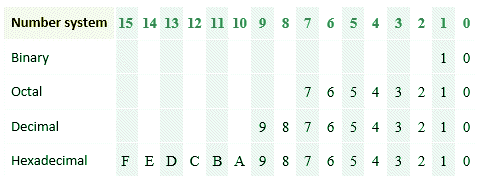Digits used by the binary, octal, decimal and hexadecimal number systems

## Decimal numbers

It is generally assumed that the use of a number system based on ten symbols (a decimal number system) is based on the fact that early man first learned to count using the fingers and thumbs on both hands, allowing them to count up to ten with relative ease.

The number systems we are interested in (including the decimal number system most of us are familiar with), are all positional systems. Consider the number 123.456 as an example. The number is a floating-point number, i.e. it has a fractional part represented by numerals that appear to the right of the decimal point (the term "floating point" simply means that the decimal point (or radix point) can be placed anywhere relative to the significant digits of the number).

The numeral in the first position before the decimal point (3) represents the value 3×10 0 (three multiplied by ten to the power zero), which equates to three, since any number to the power of zero is one. The numeral in the second position to the left of the decimal point (2) represents the value 2×10 1, or 20 (any number to the power of one is itself). The numeral in the third position to the left of the decimal point (1) represents the value 1×10 2, or one hundred.Each numeral in a decimal number must be multiplied by ten to a power that depends on the position of the numeral within the number, relative to the decimal point that separates the integer part of the number from the fractional part (if any). The position is significant, because it specifies the magnitude of the value represented by each numeral. The three numerals to the right of the decimal point in the above example (4, 5 and 6) represent the values 4×10 -1, 5×10 -2, and 6×10 -3 respectively.

## Octal numbers

The octal number system, as the name suggests, has a base of eight (8). It uses the same first eight digits as the decimal number system (0 to 7). The octal number system is no longer widely used in computing (or anywhere else for that matter) but it was popular at one time because all of the octal digits could be represented using just three bits.

Some early mainframe and minicomputer systems were built around a thirty-six-bit architecture. Octal was often used to store digital information because groupings of three bits fit rather nicely into a thirty-six-bit word. The octal digits and their binary representations are shown below.

0 = 000
1 = 001
2 = 010
3 = 011
4 = 100
5 = 101
6 = 110
7 = 111

Like the hexadecimal and binary number systems used in modern computers, octal numbers were often required to represent decimal values. We saw above what the number 123.456 represents in a decimal number system. Each digit represents some multiple of a power of ten, depending on its position relative to the radix point. What would this same sequence of numbers represent if, instead of a decimal number system we were using an octal number system? Let’s find out.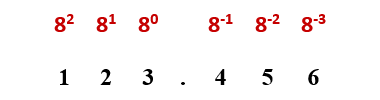To convert this value to its decimal equivalent, we need to add up the values of the digits:

123.4568   =  1 × 82 + 2 × 81 + 3 × 80 + 4 × 8-1 + 5 × 8-2 + 6 × 8-3

=  64 + 16 + 3 + 0.5 + 0.078125 + 0.01171875

=  83.58984375

As you can see, this sequence of digits represents a very different value in octal. Note that when we write a decimal number, we do not usually specify explicitly that the number is a decimal number. When writing numbers in other bases, however, it is sometime a good idea to indicate the number base used in order to avoid any confusion. We can do this by adding a subscript after the number as follows:

123.4568

We have seen that converting numbers from octal to decimal is relatively straightforward. Converting numbers from octal to binary is even easier. We have seen that each digit in an octal number is multiplied by a power of eight, and eight is equal to two to the power of three:

8 = 2 3

Each additional digit as we move from right to left in a binary number represents a successive power of two. Similarly, each additional digit as we move from right to left in an octal number represents a successive power of eight. This means that shifting one place to the left in an octal number is equivalent to shifting three places to the left in a binary number.

The significance may not be immediately obvious - you might have to think about it for a while. The important thing to realise is that in order to convert an octal number to its binary equivalent, we simply replace each octal digit with the three binary digits that represent it (we saw these listed above). Let's convert the octal number 123.456 to binary: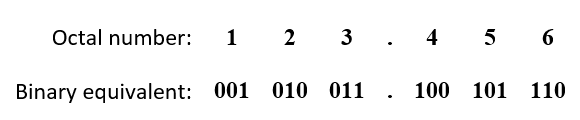Therefore:

123.4568   =  1010011.100101112

Note that we have removed the two leading zeros and the trailing zero from the binary result. You should be able to see that the reverse process (converting binary numbers to their octal representation) is simply a matter of replacing each group of three binary digits with its octal representation. Let's convert the binary number 111110101.10001101 to octal: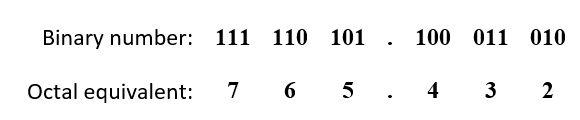Therefore:

111110101.100011012   =  765.4328

The only thing you need to be careful about here is to make sure you break the binary number down into the correct three-digit groupings (if necessary, add leading or trailing zeros to complete the left and right-most groups of binary digits.

Converting octal numbers to hexadecimal cannot be done directly – it is a two-step process. The first step is to convert the octal number to its decimal equivalent, as shown above. The second step is to convert the resulting decimal number to hexadecimal (see below).

The hexadecimal (or base sixteen) number system is a positional number system that represents numeric values using sixteen symbols - 0 to 9 for the values zero to nine, and A, B, C, D, E and F to represent the numbers ten to fifteen. For this reason, it is said to have a radix of sixteen. Hexadecimal numbers are expressed as a series of one or more hexadecimal digits, followed by a lower-case "h" or occasionally by the subscript 16 to indicate that they are in fact hexadecimal (base sixteen) numbers. Here is the decimal number one hundred and sixty-five (165) expressed as a hexadecimal number:

A5h

Like the decimal system with which we are familiar, the position of each digit within a hexadecimal number determines its value. Whereas each position in a decimal number represents some power of ten, however, each position in a hexadecimal number represents a power of sixteen. The table below shows the numbers 0 to 63 (to base ten) as hexadecimal numbers.

HexDecHexDecHexDecHexDec
00101620323048
11111721333149
22121822343250
33131923353351
44142024363452
55152125373553
66162226383654
77172327393755
88182428403856
99192529413957
A101A262A423A58
B111B272B433B59
C121C282C443C60
D131D292D453D61
E141E302E463E62
F151F312F473F63

The decimal number thirty-nine (39) expressed as a hexadecimal number (27h) is therefore:

2 × 16 1 + 7 × 16 0  =  32 + 7  =  39

The hexadecimal digit in the right-most position in any hexadecimal whole number holds the smallest value (with a maximum value of fifteen). The left-most hexadecimal digit has a maximum value that reflects its position relative to the right-most digit, and will be a multiple of some power of sixteen greater than zero. The value of the left-most hexadecimal digit (assuming that we ignore leading zeros) always exceeds the combined value of all the digits to the right of it. For this reason, the overall magnitude of a hexadecimal number is always determined by the position of the left-most digit.

Fractions can also be represented using hexadecimal digits, as can real (fractional) numbers. Like real numbers to base ten, the fractional part of a hexadecimal number follows a period. In the decimal number system, we call this a decimal point. In the hexadecimal number system, we call it a hexadecimal point.

In the decimal system, the numeral in the first position following the decimal point is multiplied by 10 -1 (0.1), the digit in the second position following the decimal point is multiplied by 10 -2 (0.01), and so on. The fractional part of a hexadecimal number works on the same principle, except that the digit in the first position following the binary point is multiplied by 16 -1 (0.0625 to base ten), the digit in the second position following the binary point is multiplied by 16 -2 (0.00390625 to base ten), and so on.

Note that, whereas each successive positive power of sixteen has sixteen times the value of its predecessor, each successive negative power of sixteen has one sixteenth the value of its predecessor. The anatomy of a real hexadecimal number is illustrated below.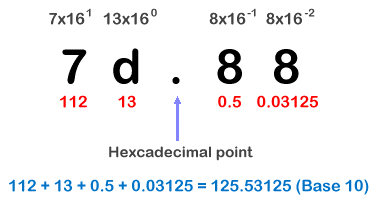The anatomy of a real hexadecimal number

Because it is so easy to represent groups of four binary digits using hexadecimal digits, the hexadecimal number system is frequently used to represent binary values in the fields of computing and digital electronics. Byte values, which can represent decimal numbers in the range 0 to 255, are often expressed using a pair of hexadecimal digits in the range 00h to FFh. Hexadecimal numbers are also commonly used to represent memory addresses in assembly language programs.

## Binary numbers

The importance of the binary number system, which as the name suggests has only two symbols (0 and 1) is that it is the only number system that modern digital computers actually "understand". It should be remembered that at the heart of these devices is a central processing unit (CPU) that is essentially a finite state machine consisting of transistors and micro-circuitry that provides hundreds of millions of interconnected high-speed switches. The state of each individual switch can be either on or off, so each switch can only represent a one or a zero.

Although computers can process huge amounts of data and carry out millions of calculations per second, the underlying machine operations that achieve this are all based on the manipulation of binary values using various types of logic circuit. Even the data stored in working memory (RAM) and on magnetic or optical disks is physically stored as binary data – billions of individual binary digits ("bits"), all having the value 0 or 1.

While integer values in other number bases can be represented exactly using a binary number system, many real numbers (those with fractional values) cannot. Such values are therefore approximated, although the degree of precision achieved increases with the number of bits used in their representation, at the cost of requiring more storage space in working memory or on disk.

Because the binary (or base two) number system represents numeric values using just two symbols (0 and 1) it is said to have a radix of two. Binary numbers are expressed as a series of binary digits, occasionally followed by a subscripted 2 to indicate that they are, in fact, binary numbers. Here is the decimal number one hundred and seventy (170) expressed as a binary number:

101010102

Like the decimal system with which we are familiar, the position of each digit within a binary number determines its value. Whereas each position in a decimal number represents some power of ten, however, each position in a binary number represents a power of two. The table below shows the numbers 0 to 15 (to base ten) as 4-bit binary numbers (fifteen is the largest number that can be expressed using four binary digits).

Binary Numbers
BinaryDecimalBinaryDecimal
0000010008
0001110019
00102101010
00113101111
01004110012
01015110113
01106111014
01117111115

The decimal number 13 would be expressed as a binary number as follows: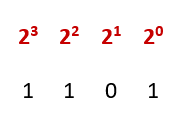1 × 2 3 + 1 × 2 2 + 0 × 2 1 + 1 × 2 0  =   8 + 4 + 0 + 1  =  13

The binary digit in the right-most position in any binary whole number holds the smallest value (with a maximum value of one) and is sometimes referred to as the least significant bit (LSB). The left-most binary digit has a maximum value that reflects its position relative to the LSB, and will be some power of two greater than zero.

The left-most binary digit (assuming that we ignore leading zeros) is sometimes referred to as the most significant bit (MSB), and its value always exceeds the combined value of all the bits to the right of it. For this reason, the overall magnitude of a binary number is always determined by the position of the left-most (non-zero) bit.

Fractions can also be represented using binary digits, as can real (fractional) numbers. Like real numbers to base ten, the fractional part of a binary number follows a radix point. In the decimal number system, we call this a decimal point, but in the binary number system we call it a binary point.

In the decimal system, the numeral in the first position following the decimal point is multiplied by 10 -1 (0.1), the digit in the second position following the decimal point is multiplied by 10 -2 (0.01), and so on. The fractional part of a binary number works on the same principle, except that the digit in the first position following the binary point is multiplied by 2 -1 (0.5 to base ten), the digit in the second position following the binary point is multiplied by 2 -2 (0.25 to base ten), and so on.

Note that, whereas each successive positive power of two has double the value of its predecessor, each successive negative power of two has half the value of its predecessor. The anatomy of a real binary number is illustrated below.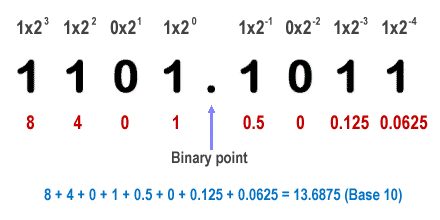The anatomy of a real binary number

It can be seen from the above that converting binary numbers to decimal is relatively straightforward. Simply add together the powers of two represented by each binary digit (this works for binary integers, fractions, and real numbers).

Almost as obvious, when you think about it, is the fact that the precision with which real numbers and fractions (to base ten) can be converted to binary will often depend on the number of bits available to represent the fractional part of the number. The greater the number of bits used, the more accurate the result will be (in computing terms, greater accuracy equates to more memory space required to store the result).

Converting binary numbers to hexadecimal is even easier to do, and allows us to represent binary values in a much more human-friendly way. We have already seen a table containing decimal integer (whole number) values from zero to fifteen (0–15) and their binary equivalents. Let's now look at the binary equivalents of the sixteen digits used by the hexadecimal system (0–9, A–F):

Binary to Hexadecimal Conversion
0000010008
0001110019
001021010A
001131011B
010041100C
010151101D
011061110E
011171111F

We have seen that each digit in a hexadecimal number is multiplied by a power of sixteen, and sixteen is equal to two to the power of four:

16  =  2 4

Each additional digit as we move from right to left in a binary number represents a successive power of two. Similarly, each additional digit as we move from right to left in a hexadecimal number represents a successive power of sixteen. This means that shifting one place to the left in a hexadecimal number is equivalent to shifting four places to the left in a binary number.

The significance may not be immediately obvious - you might have to think about it for a while. The important thing to realise is that in order to convert a binary number to its hexadecimal equivalent is simply a matter of replacing each group of four binary digits with its hexadecimal representation. Let's convert the binary number 110111110101.100011010011 to hexadecimal:Therefore:

110111110101.1000110100112   =  DF5.8D3h

The only thing you need to be careful about here is to make sure you break the binary number down into the correct four-digit groupings (if necessary, add leading or trailing zeros to complete the left and right-most groups of binary digits. Incidentally, if you want to check any of the conversion examples given on this page, you can find a handy online conversion tool here:

Converting from hexadecimal to binary is just a matter of reversing the process. In other words, we simply replace each digit in the hexadecimal number with the four binary digits that represent it. Once we have carried out the conversion, we can safely remove any leading or trailing zeros. Let's convert the hexadecimal number F08.7A5h to binary:Therefore:

F08.7A5h = 111100001000.0111101001012

## Decimal to octal conversion

One method that can be used to convert a decimal number to its octal equivalent is to carry out integer division using eight as the divisor. The number to be converted is divided by eight, and the remainder is written in the right-most position (as an octal digit). The integer result of the division is then divided by eight again, and the remainder is written (again as an octal digit) to the left of the octal digit obtained in the previous step. This process is repeated until the integer result of the division is zero. An example will serve to illustrate the process. To convert the decimal number 16,895 to octal, proceed as follows:

Decimal to Octal Conversion
Integer divisionInteger resultRemainderOctal
16,895 ÷ 82,111778
2,111 ÷ 8263778
263 ÷ 832778
32 ÷ 84008
4 ÷ 80448

Therefore:

16,89510   =  407778

We can carry out a relatively simple process - one we have already seen, in fact - to convert the number back to decimal (and at the same time confirm that the answer we obtained is correct). Simply multiply each hexadecimal digit by the appropriate power of sixteen and add the resulting decimal values together, as follows:

4 × 8 4 + 0 × 8 3 + 7 × 8 2 + 7 × 8 1 + 7 × 8 0

=  4 × 4,096 + 0 × 512 + 7 × 64 + 7 × 8 + 7 × 1

=  16,384 + 0 + 448 + 56 + 7

=  16,89510

## Decimal to hexadecimal conversion

One method that can be used to convert a decimal number to its hexadecimal equivalent is to carry out integer division using sixteen as the divisor. The number to be converted is divided by sixteen, and the remainder is written in the right-most position (as a hexadecimal digit). The integer result of the division is then divided by sixteen again, and the remainder is written (again as a hexadecimal digit) to the left of the hexadecimal digit obtained in the previous step. This process is repeated until the integer result of the division is zero. An example will serve to illustrate the process. To convert the decimal number 13,337 to hexadecimal, proceed as follows:

Decimal to Hexadecimal Conversion
Integer divisionInteger resultRemainderHex
13,337 ÷ 1683399h
833 ÷ 165211h
52 ÷ 16344h
3 ÷ 16033h

Therefore:

13,33710   =  3419h

We can carry out a relatively simple process - one we have already seen, in fact - to convert the number back to decimal (and at the same time confirm that the answer we obtained is correct). Simply multiply each hexadecimal digit by the appropriate power of sixteen and add the resulting decimal values together, as follows:

3 × 16 3 + 4 × 16 2 + 1 × 16 1 + 9 × 16 0

=  3 × 4096 + 4 × 256 + 1 × 16 + 9 × 1

=  12,288 + 1,024 + 16 + 9

=  13,33710

## Decimal to binary conversion

Converting decimal numbers to binary is not quite so straightforward, but it is not all that difficult. To make things easier, we will deal with whole numbers and fractions (or the whole number and fractional parts of real numbers) separately. Let’s start with binary integers ("integer" is the name we use for a whole number). The method described here is one of several that can be used, but is probably the most straightforward. To convert a decimal number n to binary, proceed as follows:

1. Divide n by two, and write down the remainder (zero or one).
2. If the result of the previous step is greater than zero, divide it by two and write down the remainder. Otherwise, go to step 4.
3. Repeat step 2.
4. Write down the sequence of remainder values in reverse order.

The following example should clarify this process. We are going to convert the decimal number one hundred and sixty-nine (169) to its binary equivalent.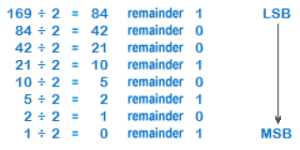Decimal-to-binary integer conversion

The binary number is read from the remainder column starting with the bottom-most bit (the most significant bit, or MSB) and ending with the top-most bit (the least significant bit, or LSB), giving us a binary value of:

16910   =  101010012

Converting decimal fractions to binary is a similar process. As with the integer conversion described above, the method shown below is not the only one available, but is relatively easy to use. It can be used for fractional values expressed either as proper fractions (e.g.  3/4 ) or decimal fractions (e.g. 0.75). To convert a decimal fraction to binary, proceed as follows:

1. Start by writing the binary point, preceded by a zero.
2. Multiply the fractional value by two.
3. If the result is less than one, add a zero.
4. If the result is one exactly, add a one and go to step 7.
5. If the result is greater than one, add a one and discard the integer part of the result to create a new fractional value.
6. Go to step 2.
7. Stop.

The following example illustrates how the process works. We are going to convert the fraction  1/3  to its binary equivalent.Decimal-to-binary conversion of a fractional value

It should quickly become apparent that the decimal fraction being converted is in this case represented by a repeating binary pattern. The precision of the conversion will therefore depend upon how many bits are available to hold the fractional value. In this case, we have allowed six bits to the right of the binary point, giving us a binary value of:

0.0101012

Note that  1/3  expressed as a decimal fraction is 0.333 recurring, and the accuracy of the decimal representation is thus dependent on the number of binary digits appearing after the radix point. When carrying out calculations involving fractional values, therefore, it is always a good idea to determine the degree of accuracy required for the answer. For binary calculations, there will usually be some upper limit on the number of bits available for the fractional part of the result.